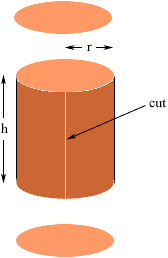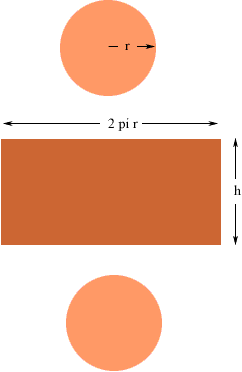Quandaries and Queries Who is asking: Parent Level: Middle Question: How do you find the radius of a cylinder when you are given only the surface area and the height of the cylinder? Hi Kathryn, Think about the reverse. If you had all the information you needed, how would you find the surface area of a cylinder. I am going to do this assuming that the surface area of the cylinder includes the ends. If the surface area doesn't include the ends it is earier. I think of a cylinder as a soup can. Use your can opener and cut off the ends and then cut vertically up the cylindrical piece that remains and roll it out flat. I have labeled the radius of the cylinder and its height.This gives you three pieces, two circular disks and a rectangle.The disks each have area pi r2 and the rectangle has area 2 pi r h Thus surface area = 2 pi r2 + 2 pi r h If you know the surface area and the height of the cylinder substitute these values and you have a quadratic equation for r. If your value for the surface area does not include the ends then you have surface area = 2 pi r h which is a linear equation for r. I hope this helps, Penny Go to Math Central